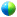18th Conference on Probability and Statistics in the Atmospheric Sciences

1.6

Extending ROC analysis to the multicategory forecast problem

Matthew S. Wandishin, Univ. of Arizona and NSSL, Tucson, AZ; and S. L. Mullen

Receiver operating characteristic (ROC) analysis has become an established tool for evaluating forecast discrimination, that is, the ability of a forecast system to distinguish between ‘yes' events and ‘no' events. However, not all forecast problems involve such a simple binary situation. For example, the Climate Prediction Center (CPC) issues seasonal forecasts for temperature and precipitation as probabilities that conditions will fall into one of three categories: above normal, normal, or below normal.

One difficulty with extending ROC analysis to multiclass problems is dimensionality. A ROC curve plots a series of true- and false-positive rate (in the meteorological literature these are sometimes referred to as the probability of detection and probability of detection of false detection, respectively) pairs obtained by varying the critical decision threshold forecast probability, but the true-positive rate (TPR) is just 1 – FNR (false negative rate). Thus it is seen that the points along a ROC curve are tied to the misclassification information (i.e., the false-positive and false-negative rates), that is, the off-diagonal elements of the standard 2 x 2 verification table. For the binary problem, the number of off-diagonal elements equals the number of diagonal elements and leads to a simple two-dimensional curve. But for the three-class problem, there are six off-diagonal elements, creating a graphically challenging situation. More generally, for the n-class problem the number of off-diagonal elements, and thus the dimensionality of the ROC graph, is n*(n-1).

Drawing from work in the medical and machine learning communities, the extension of the ROC curve to the multiclass problem is shown, along with estimates to the area (volume) beneath the ROC curve (surface) obtained from efforts to make the high-dimensional problem more manageable. The multi-class ROC will be illustrated with a simple artificial data set as well as 10 years of CPC seasonal temperature and precipitation forecasts.Recorded presentation

Session 1, Forecast Evaluation
Monday, 30 January 2006, 9:00 AM-11:45 AM, A304

Previous paper  Next paper

Browse or search entire meeting